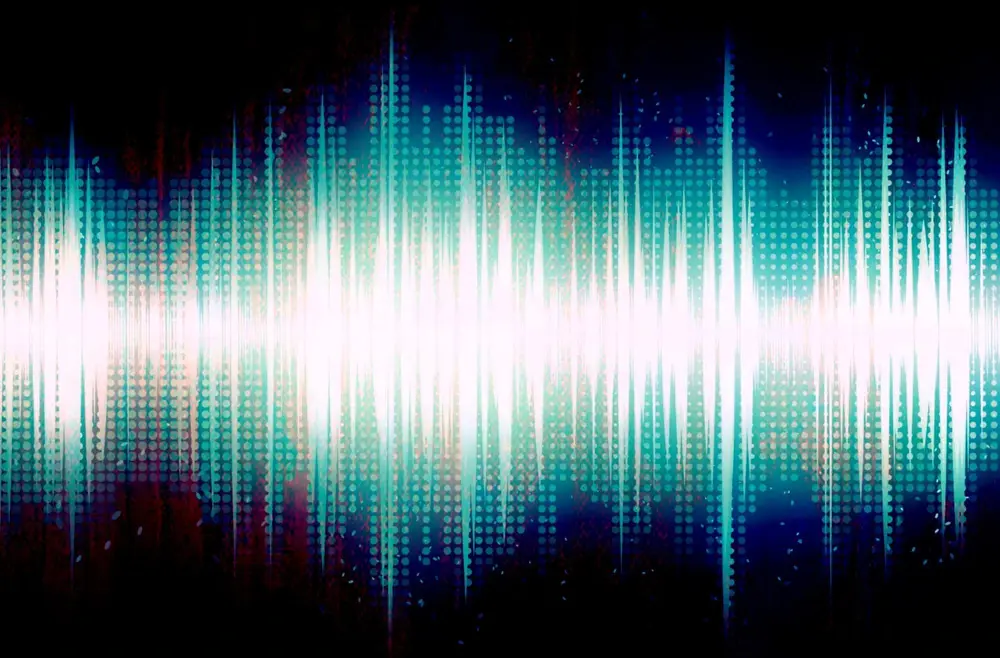# Waves

Waves do not carry matter but they transfer energy.

There are two main types of wave: Transverse Waves and Longitudinal Waves. Below is some information and examples of both:

#### Transverse waves:

• A transverse wave moves across the direction in which the wave is travelling.
• An example of a transverse wave is the sea - imagine the shape of a wave.
• Another good example is a light wave.

#### Longitudinal waves:

• Longitudinal waves look like this: ||| | | || || | |||| | | | ||
• They have a series of compressions and rarefractions.
• A compression is when the wavelength (distance between each wave) is small.
• A rarefraction is when the wavelength is large.
• Longitudinal waves pass on energy as a series of vibrations.
• These are sound waves.
• Wavelength. The wavelength is the length of a wave e.g. the distance between one crest (high part) and the next crest.
• Frequency. Measure of how many waves pass in one second.
• Amplitude. The distance between the crest of the wave and the centre line.
• Formulae. There are two main formulae on the topic of waves:
• Velocity (m/s) = frequency (Hz) x wavelength (m)
• Speed (m/s) = distance (m) / time (s)
Waves

Waves

Waves